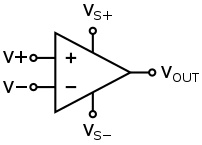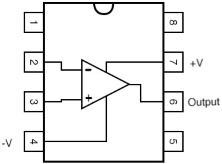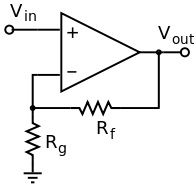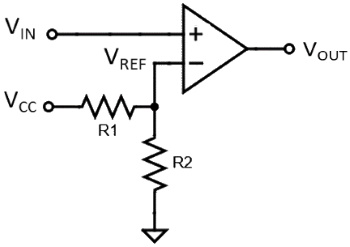#Operational Amplifier by Stephen BucaroOver 1 million movies and TV episodesop amp schemtaic sysmbol

An operational amplifier (more often referred to as an op amp) is an amplifier circuit with a differential input, in other words it amplifies the voltage difference between its two inputs. An op amp that has a very high gain, typically 100,000, but is usually configured for a lower gain.op amp in 8-pin DIP package

Op amps come in several different packages, but the 8-pin DIP package is the most popular. The op amp requires a positive (V+) supply voltage and a negative (V? supply voltage). It has a non-inverting input (+), and an inverting input (?), and it amplifies the difference in voltage between the two, called the differential voltage.op amp with negative feedback

The open-loop gain of an op amp (open-loop referring to operation without an external feedback loop) is typically very large and so even a quite small difference between the V+ and V? inputs drives the amplifier into saturation. Normally an op amp would not be used open-loop. To reduce the gain, negative feedback is used as shown above.

With negative feedback, the circuits voltage gain would be:

G = 1 + Rf/Rgop amp configured as a comparator

An op amp might be used open-loop, or in a high-gain configuration when used as a comparator. As a comparator, the op amp compares the voltage level on one input with another voltage level set by the voltage divider circuit on the other input, as shown above. When the voltage on the input is below the voltage set by the divider, the op amp output stays at V-. When the voltage on the input raises above the voltage at the divider, the op amp output rapidly rises to V+.